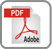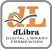# ON THE NUMERICAL SOLUTION OF SINGULAR INTEGRAL EQUATIONS USING SANDIKIDZE’S APPROXIMATON

1 Department of Mathematics, University of M’sila, 2800 M’sila, Algeria
2InstituteofApplied Mathematics, Tbilisi, Georgia

Rec. 19 March 2004

### Abstract:

The aim of this work is to solve singular integral equations (S.I.E), of Cauchy type on a smooth curve by pieces. This method is based on the approximation of the singular integral of the dominant part , where the (S.I.E) is reduced to a linear system of equations and to realize this approach numerically by the means of a program [3, 5].

### References:

 D. J. Antidze, On the approximate solution of singular integral equations, Seminar of Institute of Applied Mathematics, 1975, Tbilissi.
 N. I. Muskhelishvili, Singular integral equations, “Nauka” Moscow, 1968, English transl, of lsted Noordhoff, 1953; reprint,1972.
 M. Nadir, Problemes aux limites qui se reduisent aux equations integrales de Fredholm, Seminaire de l’Institut de Mathematiques et Informatique, 1985, Annaba.
 M. Nadir, Operateurs integraux et bases d’ondelettes, Far East J. Sci. 6(6) 977-995 (1998).
 M. Nadir, On the approximation of theHilbert transform, Far East J. App. Math. 1, 71-786 (2003).
 J. Sanikidze, On approximate calculation of singular line integral, Seminar of Institute of Applied Mathematics, 1970, Tbilissi
 J. Sanikidze, Approximate solution of singular integral equations in the case of closed contours of integration, Seminar of Institute of Applied Mathematics, 1971, Tbilissi.